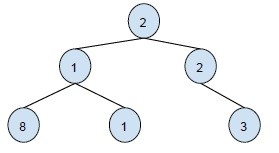# Print BST keys in the given range in C++

C++Server Side ProgrammingProgramming

In this problem, we are given two nodes of a binary search tree. And we have to print all the values in the range of k1 to k2 that occur in the tree. That is we have to print all the values that are greater than k1 and smaller than k2. And we have to print all these keys in increasing order of their values.

Binary Search Tree is a tree which follows these 3 properties −

• The left subtree has nodes with values lesser than the node’s value.

• The right subtree has a node with values greater than the node’s value.

• A subtree’s taken should also be a binary search tree. No duplicate nodes are allowed in the tree.

Example,Let’s take an example to understand the topic better,

Input : k1 = 12 and k2 = 25.
Output : 15, 20, 24

Tree -Explanation − On traversing the tree, all elements will be traversed and the elements that lie between the range 12 to 25 i.e. for a node x, 12 ≤ x ≥ 25 will be printed.

Here, we will use the properties of BST to find our solution. i.e. all elements in the left subtree are smaller than root and all elements in the right subtree are greater than the root node. And we will use in order traversal of the BST to solve this problem. Now, let's create an algorithm to solve this problem.

## ALGORITHM

Step 1 : Compare the root node with the k1 and k2.
Step 2 : If root is greater than k1. Call left subtree for the search recursively.
Step 3 : If root is smaller than k2. Call right subtree for the search recursively.
Step 4 : If the root of the tree is in the range. Then print the root’s value.

## Example

Now, based on this algorithm, let’s create a program to solve the problem.

#include<bits/stdc++.h>
using namespace std;
class node{
public:
int data;
node *left;
node *right;
};
void nodesInRange(node *root, int k1, int k2){
if ( NULL == root )
return;
if ( k1 < root->data )
nodesInRange(root->left, k1, k2);
if ( k1 <= root->data && k2 >= root->data )
cout<<root->data<<"\t";
if ( k2 > root->data )
nodesInRange(root->right, k1, k2);
}
node* insert(int data){
node *temp = new node();
temp->data = data;
temp->left = NULL;
temp->right = NULL;
return temp;
}
int main(){
node *root = new node();
int k1 = 12, k2 = 25;
root = insert(20);
root->left = insert(10);
root->right = insert(24);
root->left->left = insert(8);
root->left->right = insert(15);
root->right->right = insert(32);
cout<<”The values of node within the range are\t”;
nodesInRange(root, k1, k2);
return 0;
}

## Output

The values of node within the range are 15 20 24.